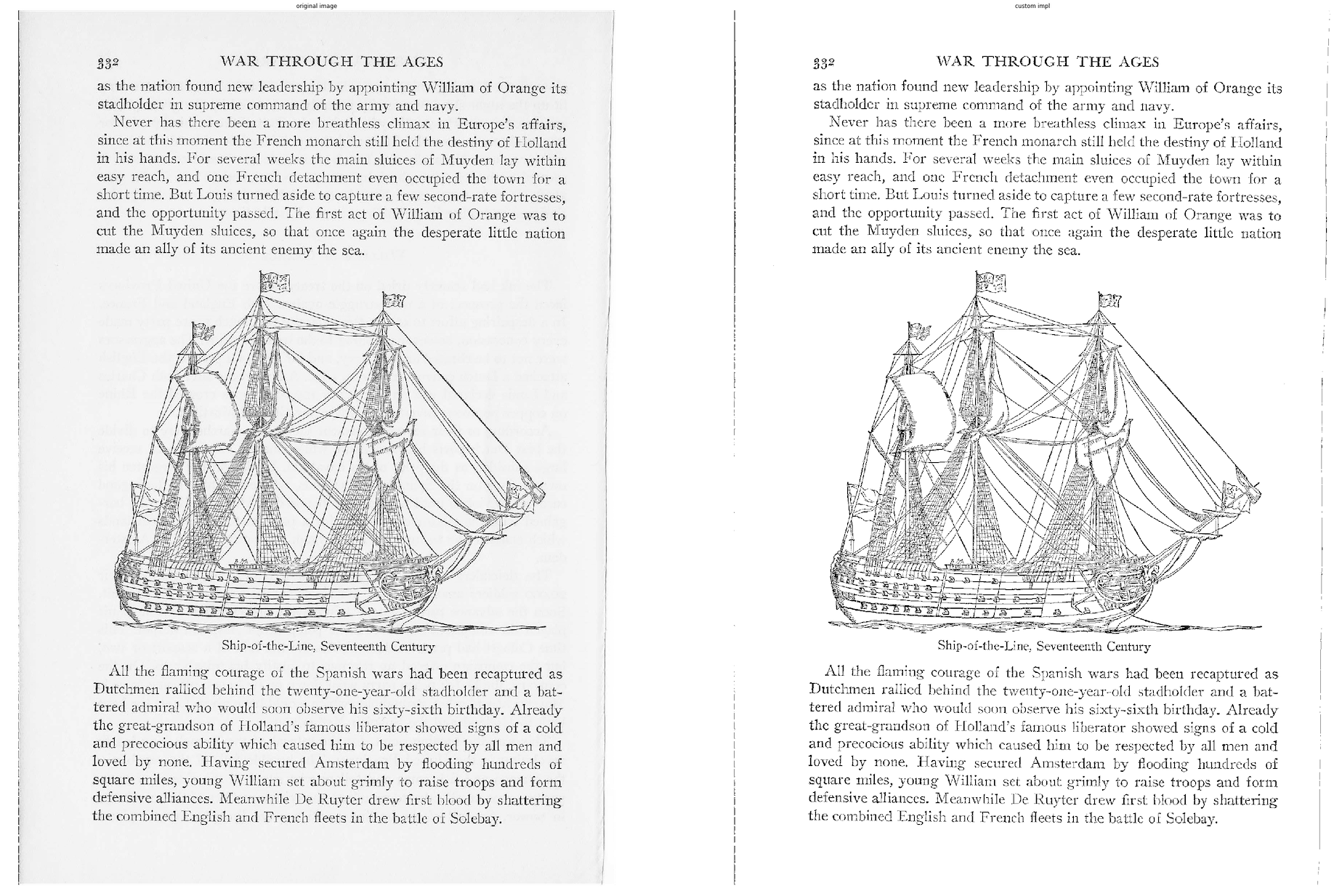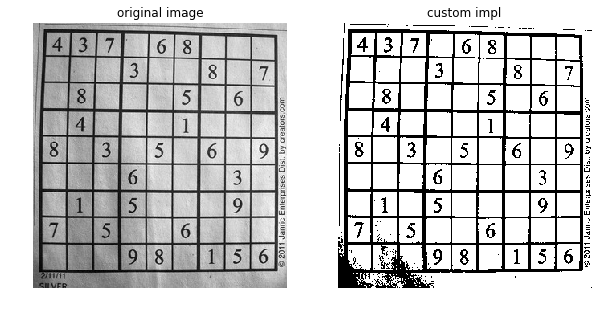# Otsu’s method for image thresholding explained and implemented

The process of separating the foreground pixels from the background is called thresholding. There are many ways of achieving optimal thresholding and one of the ways is called the Otsu’s method, proposed by Nobuyuki Otsu. Otsu’s method is a variance-based technique to find the threshold value where the weighted variance between the foreground and background pixels is the least. The key idea here is to iterate through all the possible values of threshold and measure the spread of background and foreground pixels. Then find the threshold where the spread is least.

## Algorithm

The algorithm iteratively searches for the threshold that minimizes the within-class variance, defined as a weighted sum of variances of the two classes (background and foreground). The colors in grayscale are usually between 0-255 (0-1 in case of float). So, If we choose a threshold of 100, then all the pixels with values less than 100 becomes the background and all pixels with values greater than or equal to 100 becomes the foreground of the image.

The formula for finding the within-class variance at any threshold t is given by:

$$\sigma ^2 (t) = \omega_{bg}(t) \sigma_{bg} ^2 (t) + \omega_{fg}(t) \sigma_{fg} ^2 (t)$$.   $$(1)$$

where $$\omega_{bg}(t)$$ and $$\omega_{fg}(t)$$ represents the probability of number of pixels for each class at threshold t and $$\sigma ^2$$ represents the variance of color values.

To understand what this probaility means, Let,

$$P_{all}$$ be the total count of pixels in an image,
$$P_{BG}(t)$$ be the count of background pixels at threshold t,
$$P_{FG}(t)$$ be the count of foreground pixels at threshold t

So the weights are given by,

$$\omega_{bg}(t) = \frac{P_{BG}(t)}{ P_{all}}$$

$$\omega_{fg}(t) = \frac{P_{FG}(t)}{ P_{all}}$$

The variance can be calculated using the below formula:

$$\sigma ^2 (t) = \frac{ \sum (x_{i}-\overline{x} )^{2} }{N-1}$$

where,

$$x_{i}$$ is the value of pixel at i in the group (bg or fg)
$$\overline{x}$$ is the means of pixel values in the group (bg or fg)
$$N$$ is the number of pixels.

Now, Let’s understand the formula by finding the within-class variance at one threshold, T=100

For the above image, at T=100,  we will get the background and foreground as shown below:

Here,
$$P_{all} = 16$$
$$P_{BG} = 7$$
$$P_{FG} = 9$$

Our weights will be,

$$\omega_{bg}(t) = \frac{P_{BG}(t)}{ P_{all}} = 7/16 = 0.44$$
$$\omega_{fg}(t) = \frac{P_{FG}(t)}{ P_{all}} = 9/16 = 0.56$$

Now to find the variance, we find the mean first.

$$\overline{x_{bg}} = \frac{21+22+25+26+27+23+24}{7} = 24$$
$$\overline{x_{fg}} = \frac{120+120+160+180+190+123+145+165+175}{9} = 153.1$$

The variances are given by,

$$\sigma_{bg} ^2 (t=100)= \frac{(21-24)^2+(22-24)^2…….(24-24)^2}{7} = 4.0$$
$$\sigma_{fg} ^2 (t=100)= \frac{(120-153.1)^2+(120-153.1)^2…….(175-153.1)^2}{9} = 657.43$$

Substituting everything in equation (1) we get,

$$\sigma ^2 (t=100) = 0.44 * 4.0 + 0.56 * 657.43 = 369.9208$$

Similarly, we can find for other values of t also.T=22, $$\sigma ^2 = 4092.58$$ T=23, $$\sigma ^2 = 3667.60$$ T=25, $$\sigma ^2 = 2642.35$$T=26, $$\sigma ^2 = 2009.93$$ T=28, $$\sigma ^2 = 371.55$$ T=124, $$\sigma ^2 = 1316.48$$

The value of variance remains the same from 28 and 120.

If you see the above variances, its least at T=28 or more precicely between 28 to 120.

Thus our Otsu threshold = 28.

## Python Implementation

def threshold_otsu_impl(image, nbins=0.1):

#validate grayscale
if len(image.shape) == 1 or len(image.shape) > 2:
print("must be a grayscale image.")
return

#validate multicolored
if np.min(image) == np.max(image):
print("the image must have multiple colors")
return

all_colors = image.flatten()
total_weight = len(all_colors)
least_variance = -1
least_variance_threshold = -1

# create an array of all possible threshold values which we want to loop through
color_thresholds = np.arange(np.min(image)+nbins, np.max(image)-nbins, nbins)

# loop through the thresholds to find the one with the least within class variance
for color_threshold in color_thresholds:
bg_pixels = all_colors[all_colors < color_threshold]
weight_bg = len(bg_pixels) / total_weight
variance_bg = np.var(bg_pixels)

fg_pixels = all_colors[all_colors >= color_threshold]
weight_fg = len(fg_pixels) / total_weight
variance_fg = np.var(fg_pixels)

within_class_variance = weight_fg*variance_fg + weight_bg*variance_bg
if least_variance == -1 or least_variance > within_class_variance:
least_variance = within_class_variance
least_variance_threshold = color_threshold
print("trace:", within_class_variance, color_threshold)

return least_variance_threshold

The entire notebook is here. https://github.com/muthuspark/ml_research/blob/master/Otsu%20Thresholding%20implementation.ipynb

Some sample thresholding using the Otsu Method.References:

1. “Nobuyuki Otsu (1979), A Threshold Selection Method from Gray-Level Histograms” – https://ieeexplore.ieee.org/document/4310076
2. https://en.wikipedia.org/wiki/Otsu’s_method